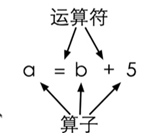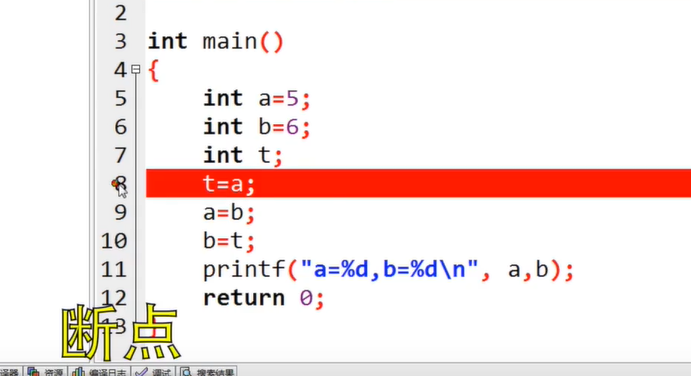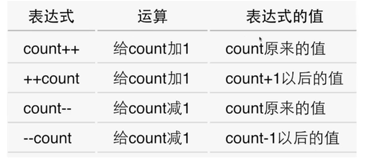# 2.1.2 变量定义

1) 有办法输入数字；
2) 有地方放输入的数字；
3) 输入的数字参与计算。

``````    int price=0;//定义了整形变量price，类型是int，初始值=0
printf("请输入金额（元）：");
int change=100-price;
printf("找您%d元。\n",change);``````

<类型名称> <变量名称>

（敲下回车之前，程序不会读到任何东西。）

# 2.1.3 变量赋值与初始化

``    int price=0;//其中=是赋值运算符，把右边的值赋给左边的变量。``

``    int a=0,b=1;``

C语言是有类型的语言，所有变量在使用前必须先定义或声明；所有变量必须有确定的数据类型（表示在变量中可以存放什么样的数据），变量中也只能存放指定类型的数据，程序运行过程中也不能改变变量的类型。
`int charge=100-price;`

``````//C语言
int price=0;
printf("请输入金额（元）：");
scanf("%d",&price);
int change=100-price;
printf("找您%d元。\n",change);``````
``````//传统的ANSI C只能在开头的地方定义变量
int price=0;
int change=0;
printf("请输入金额（元）：");
scanf("%d",&price);
change=100-price;
printf("找您%d元。\n",change);``````

# 2.1.4 变量输入

`scanf()`函数，读到的结果赋值给后面的变量
（注意变量前的&）

# 2.1.5 常量vs变量

``    const int amount=100;``

（一般const的变量全大写）
scanf的空格有讲究，以后再说。输入时如果要两个数，在中间、最后敲空格或回车，计算机读到两个数字时停止

# 2.1.6 浮点数

C语言中两个整数做运算得到的结果也只能是个整数（去掉小数部分）。而10和10.0在c中是完全不同的两个数字，10.0是浮点数（浮点数指小数点时可以浮动的，是计算机中表示分数和无理数的一种方式。人们用浮点数来称呼有小数点的数）（其实还有定点数。但是在c语言中无）

（如：

``````int a,b;
printf("a/b*3");//改进为a/b*3.0``````

(计算机里会有这种纯粹的整数，是因为运算快、占地小。而且日常生活中大多也是整数运算。)

# 2.2.1 表达式``````    int hour1,min1;
int hour2,min2;
scanf("%d %d",&hour1,&min1);
scanf("%d %d",&hour2,&min2);
int t1=hour1*60+min1;//把小时转化为分钟单位
int t2=hour2*60+min2;
int t=t2-t1;
printf("时间差是%d小时%d分。“,t/60,t%60);//t/60是小时部分；t%60取余，是分钟部分``````

# 2.2.2 运算符优先级

（double输入的时候用`%lf`，输出的时候用`%f`就行）

1 + 单目不变 自右向左 a*+b
1 - 单目取负 自右向左 a*-b
2 * 自左向右 a*b
2 / 自左向右 a/b
2 % 取余 自左向右 a%b
3 + 自左向右 a+b
3 - 自左向右 a-b
4 = 赋值 自右向左 a=b

（a+b,a-b是双目；+a,-a是单目，相当于正负号）

`a=6`的结果就是a被赋予的值，也就是6
`a=b=6`：自右向左，a=(b=6)。

``````    result=a=b=3+c;
result=(result=result*2)*6*(result=3+result);``````

（C语言没有幂次，所以只能一个个乘。）

# 2.2.3 交换变量

``````a=b;
b=a;``````

``````c=a;
a=b;
b=c;``````C语言：使用Dev C++断点调试

# 2.2.4 复合赋值

`a+=5`表示`a=a+5`(注意两个运算符之间无空格)

`a*=b+5`表示`a=a*(b+5)`,如果想先算`a=a*b`记得加括号）
INC递增、DEC递减运算符：`++``--`：单目运算符，只能有一个算子，这个算子还只能是变量（如`a++`后缀形式，`++a`前缀形式）让该变量+1/-1；
`a++`运算式的值是a+1以前的值；`++a`运算式的值是a+1以后的值。无论哪个，a的值都+1了`++``--`可以单独使用，但不要把它组合进表达式，复杂。

# 3.0.1 PAT再解释

1. 看样例数据和看题目一样重要；
2. 不要交互地输出，题目要求输出什么就输出什么，不要画蛇添足，一个空格不对都不算对）；
3. 不要检查输入，题目只会输入定义域范围内的数字；
4. 严格遵守输出的格式；
5. scanf里不要乱输什么东西（？）

`int a/b;`如果后面是小数，c语言会强制类型转换为整数。但以后学习c++或java是不可以的，要记得类型转换。

### 最后编辑：2022/8/26编辑：xuanyun